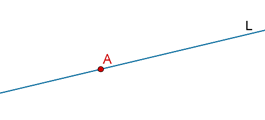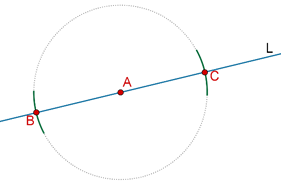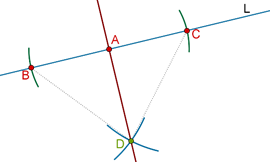# Constructing an Angle of 90°

Go back to  'Triangles-Quadrilaterals'

Suppose that you have a line L and some point A on L:How can you construct the perpendicular line to L through A? The steps of the construction are outlined below:

Step 1: Taking A as center and any radius, draw two circular arcs which intersect L on both sides of A (at B and C):Step 2: Taking B and C as centers and a radius equal to more than half of BC, draw two arcs on the same side of L, which intersect each other at D.Step 3: Draw a line through A and D. This is the required perpendicular to L.Proof: Compare $$\Delta ABD$$ and $$\Delta ACD$$:

1. AB = AC (arcs of equal radii)

2. BD = CD (arcs of equal radii)

$$\angle BAD$$ = $$\angle CAD$$ = ½ (1800) = 900